# 1512 Bigger Bites of Cake

### Using the Cake Method:

I like using the cake method to find the prime factorization of a number. I also use it to find square roots.

If you know the multiplication table well, dividing by any number from 2 to 9 is not difficult.

It is easy to check to see if a number is divisible by 4 or by 9. And it is actually easier to divide by 4 once than it is to divide by 2 twice or to divide by 9 once than it is to divide by 3 twice. That way we get to take bigger bites of cake!

I know that 1512 is divisible by 4 because the number formed from the last two digits in order, 12, is divisible by 4.

I also know that 1512 is divisible by 9 because 1 + 5 + 1 + 2 = 9.

Thus, I’ll begin by dividing first by 4 and then the result by 9 as illustrated below: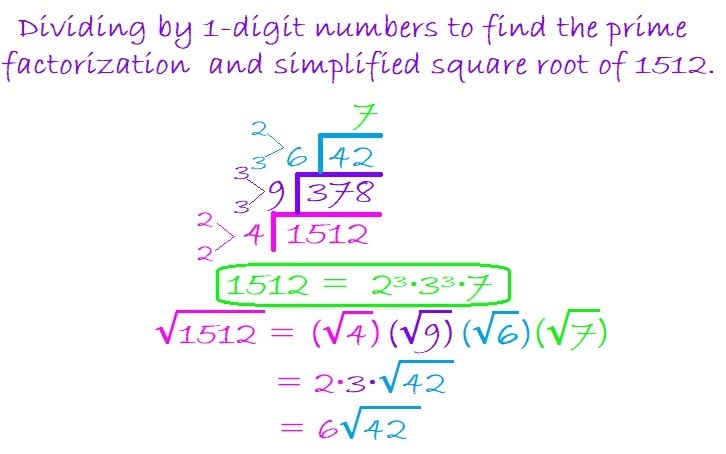When my new divisor becomes 42, if I didn’t remember where it appears in the multiplication table, I would still be fine. I know that 42 is divisible by 2 because it is even and 3 because 4 + 2 = 6, a number divisible by 3.

Thus I can divide 42 by 6 because 2 × 3 = 6, and it is far easier to divide 42 by 6 than it is to divide it first by 2 and then by 3.

To take the square root of 1512, I simply take the square root of the numbers on the outside of the cake. 4 and 9 are perfect squares so I use their square roots. Neither 6 nor 7 has any perfect squares, so I just multiply them together to get 42.

Dividing 1512 this way allowed me to make just a three-layer cake instead of a 6-layer cake to find its prime factorization and its square root!

Some people prefer to do yard work more than having cake, so here is one of the MANY possible factor trees for 1512. Make sure you pick up all seven of the leaves with prime numbers wherever they are.### Factors of 1512:

• 1512 is a composite number.
• Prime factorization: 1512 = 2 × 2 × 2 × 3 × 3 × 3 × 7, which can be written 1512 = 2³ × 3³ × 7.
• 1512 has at least one exponent greater than 1 in its prime factorization so √1512 can be simplified. Taking the factor pair from the factor pair table below with the largest square number factor, we get √1512 = (√36)(√42) = 6√42.
• The exponents in the prime factorization are 2, 1, and 2. Adding one to each exponent and multiplying we get (3 + 1)(3 + 1)(1 + 1) = 4 × 4 × 2 = 32. Therefore 1512 has exactly 32 factors.
• The factors of 1512 are outlined with their factor pair partners in the graphic below.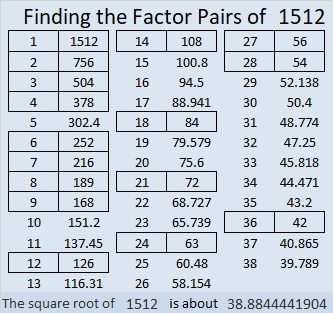# 1249 and Level 5

Level 5 puzzles can be a little tricky, but if you think about all the factors for the given clues and use logic, you should be able to solve this one. Even if the puzzle tricks you, don’t give up!Print the puzzles or type the solution in this excel file: 10-factors-1242-1250

Now I’ll share some facts about the number 1249:

• 1249 is a prime number.
• Prime factorization: 1249 is prime.
• The exponent of prime number 1249 is 1. Adding 1 to that exponent we get (1 + 1) = 2. Therefore 1249 has exactly 2 factors.
• Factors of 1249: 1, 1249
• Factor pairs: 1249 = 1 × 1249
• 1249 has no square factors that allow its square root to be simplified. √1249 ≈ 35.34119

How do we know that 1249 is a prime number? If 1249 were not a prime number, then it would be divisible by at least one prime number less than or equal to √1249 ≈ 35.3. Since 1249 cannot be divided evenly by 2, 3, 5, 7, 11, 13, 17, 19, 23, 29 or 31, we know that 1249 is a prime number.1249 is the sum of three consecutive prime numbers:
409 + 419 + 421 = 1249

1249 is the sum of two squares:
32² + 15² = 1249

1249 is the hypotenuse of a Pythagorean triple:
799-960-1249 calculated from 32² – 15², 2(32)(15), 32² + 15²

Here’s another way we know that 1249 is a prime number: Since its last two digits divided by 4 leave a remainder of 1, and 32² + 15² = 1249 with 32 and 15 having no common prime factors, 1249 will be prime unless it is divisible by a prime number Pythagorean triple hypotenuse less than or equal to √1249 ≈ 35.3. Since 1249 is not divisible by 5, 13, 17, or 29, we know that 1249 is a prime number.

# 1248 Factor Trees

It is easy to see that 1248 is divisible by 12 and therefore by 2, 3, 4, and 6, but it actually has a lot more factors than that. I decided to celebrate its many factors by making just a few of its many possible factor trees.Why does 1248 have so many factors? Well, check out its prime factorization:

• 1248 is a composite number.
• Prime factorization: 1248 = 2 × 2 × 2 × 2 × 2 × 3 × 13, which can be written 1248 = 2⁵ × 3 × 13
• The exponents in the prime factorization are 5, 1, and 1. Adding one to each and multiplying we get (5 + 1)(1 + 1)(1 + 1) = 6 × 2 × 2 = 24. Therefore 1248 has exactly 24 factors.
• Factors of 1248: 1, 2, 3, 4, 6, 8, 12, 13, 16, 24, 26, 32, 39, 48, 52, 78, 96, 104, 156, 208, 312, 416, 624, 1248
• Factor pairs: 1248 = 1 × 1248, 2 × 624, 3 × 416, 4 × 312, 6 × 208, 8 × 156, 12 × 104, 13 × 96, 16 × 78, 24 × 52, 26 × 48, or 32 × 39
• Taking the factor pair with the largest square number factor, we get √1248 = (√16)(√78) = 4√78 ≈ 35.32704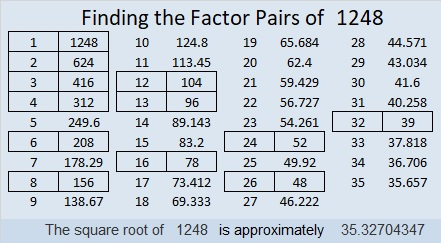1248 is the sum of four consecutive prime numbers:
307 + 311 + 313 + 317 = 1248

1248 is also the hypotenuse of a Pythagorean triple:
480-1152-1248 which is (5-12-13) times 96

# 1232 Factor Cake

1232 is divisible by 2 because it’s even.
It’s divisible by 4 because 32 is divisible by 4
It’s divisible by 8 because 232 is divisible by 8.
It also happens to be divisible by 16 and by 7.
It’s divisible by 11 because 1 – 2 + 3 – 2 = 0

1232 makes a delicious-looking factor cake:From the factor cake, we see that 2 · 2 · 2 · 2 · 7 · 11 = 1232.

Here’s more about the number 1232:

• 1232 is a composite number.
• Prime factorization: 1232 = 2 × 2 × 2 × 2 × 7 × 11, which can be written 1232 = 2⁴ × 7 × 11
• The exponents in the prime factorization are 4, 1 and 1. Adding one to each and multiplying we get (4 + 1)(1 + 1)(1 + 1) = 5 × 2 × 2 = 20. Therefore 1232 has exactly 20 factors.
• Factors of 1232: 1, 2, 4, 7, 8, 11, 14, 16, 22, 28, 44, 56, 77, 88, 112, 154, 176, 308, 616, 1232
• Factor pairs: 1232 = 1 × 1232, 2 × 616, 4 × 308, 7 × 176, 8 × 154, 11 × 112, 14 × 88, 16 × 77, 22 × 56, or 28 × 44
• Taking the factor pair with the largest square number factor, we get √1232 = (√16)(√77) = 4√77 ≈ 35.09986The odd prime factors of 1232 are 7 and 11.
OEIS.org informs us that (7 × 8 × 9 × 10 × 11) / (7 + 8 + 9 + 10 + 11) = 1232

# 1170 Factor Trees

1170 is one of those numbers with twenty-four factors. Why does it have so many? Because of its prime factorization. You can find a number’s prime factorization by making a factor tree. Here are eleven different factor trees for 1170. Each one of them produces the same prime factorization: 1170 = 2 × 3² × 5 × 13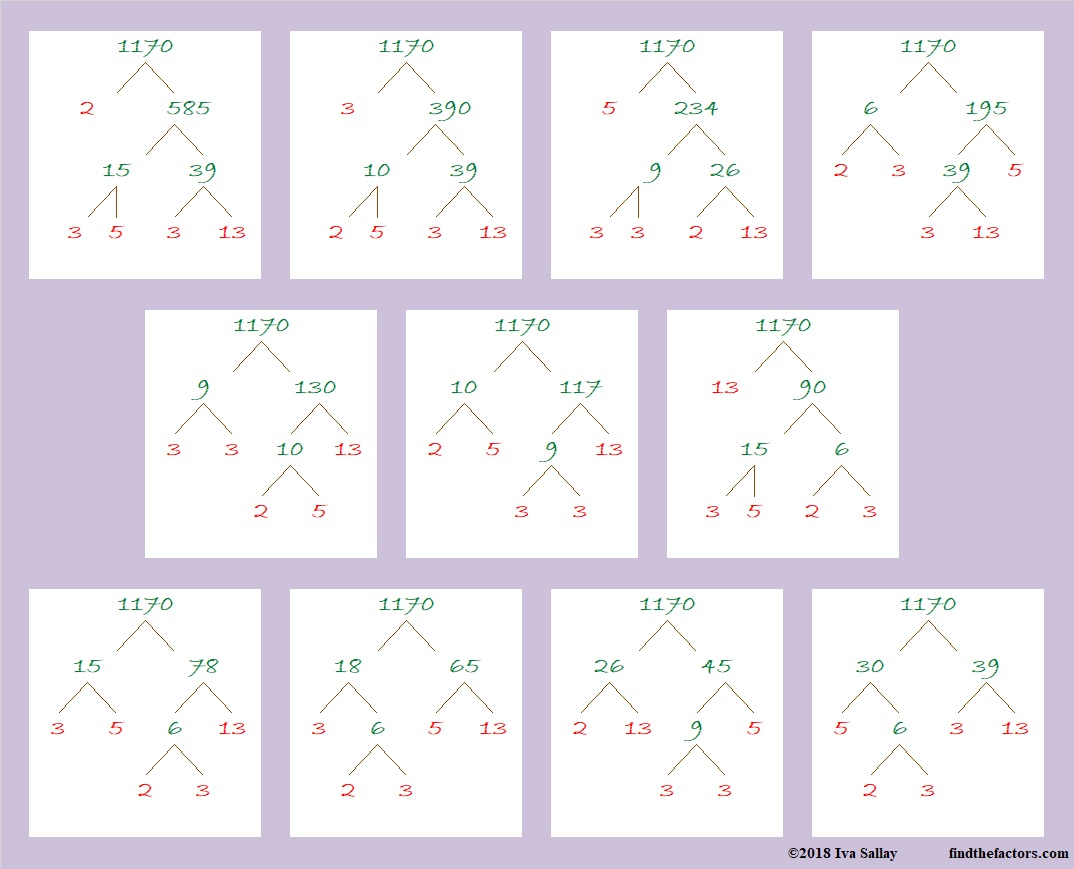• 1170 is a composite number.
• Prime factorization: 1170 = 2 × 3 × 3 × 5 × 13, which can be written 1170 = 2 × 3² × 5 × 13
• The exponents in the prime factorization are 1, 2, 1, and 1. Adding one to each and multiplying we get (1 + 1)(2 + 1)(1 + 1)(1 + 1) = 2 × 3 × 2 × 2 = 24. Therefore 1170 has exactly 24 factors.
• Factors of 1170: 1, 2, 3, 5, 6, 9, 10, 13, 15, 18, 26, 30, 39, 45, 65, 78, 90, 117, 130, 195, 234, 390, 585, 1170
• Factor pairs: 1170 = 1 × 1170, 2 × 585, 3 × 390, 5 × 234, 6 × 195, 9 × 130, 10 × 117, 13 × 90, 15 × 78, 18 × 65, 26 × 45, or 30 × 39
• Taking the factor pair with the largest square number factor, we get √1170 = (√9)(√130) = 3√130 ≈ 34.2052633² +  9² = 1170
27² +  21² = 1170

1170 is also the hypotenuse of FOUR Pythagorean triples:
288-1134-1170 calculated from 27² –  21², 2(27)(21), 27² +  21²
450-1080-1170 which is (5-12-13) times 90
594-1008-1170 calculated from 2(33)( 9), 33² –  9², 33² +  9²
702-936-1170 which is (3-4-5) times 234

1170 is 102102 in BASE 4 because 4⁵ + 2(4³) +4² + 2(1) = 1170,
and it’s repdigit 2222 in BASE 8 because 2(8³ + 8² + 8¹ + 8⁰) = 2(585) = 1170

# Reasons to Celebrate 1155

1155 is the product of the four smallest odd prime numbers. That alone is a reason to celebrate. Here, I’ve made a factor cake with 1155 for you to enjoy!make science GIFs like this at MakeaGif

Besides 1155 being the smallest number divisible by the first 4 odd prime numbers, what else can I say about it?

• 1155 is a composite number.
• Prime factorization: 1155 = 3 × 5 × 7 × 11
• The exponents in the prime factorization are 1, 1, 1, and 1. Adding one to each and multiplying we get (1 + 1)(1 + 1)(1 + 1)(1 + 1) = 2 × 2 × 2 × 2 = 16. Therefore 1155 has exactly 16 factors.
• Factors of 1155: 1, 3, 5, 7, 11, 15, 21, 33, 35, 55, 77, 105, 165, 231, 385, 1155
• Factor pairs: 1155 = 1 × 1155, 3 × 385, 5 × 231, 7 × 165, 11 × 105, 15 × 77, 21 × 55, or 33 × 35
• 1155 has no square factors that allow its square root to be simplified. √1155 ≈ 33.98529Since it’s number of prime factors is a power of 2, it can make some very well-balanced and attractive factor trees:1155 is the hypotenuse of a Pythagorean triple:
693-924-1155 which is (3-4-5) times 231

1155 is one number away from the next perfect square, AND it is the difference of two squares EIGHT different ways.
34² – 1² = 1155
38² – 17² = 1155
46² – 31² = 1155
58² – 47² = 1155
86² – 79² = 1155
118² – 113² = 1155
194² – 191² = 1155
578² – 577² = 1155

Those eight ways correspond to its eight factor pairs. Notice that both of the numbers in all of its factor pairs are odd numbers.

I like the way 1155 looks in some other bases:
It’s 3A3 in BASE 18 (A is 10 base 10) because 3(18²) + 10(18) + 3(1) = 1155
XX in BASE 34 (X is 33 base 10) because 33(34) + 33(1) = 33(35) = 1155
X0 in BASE 35 because 33(35) = 1155

XX X0 . . . Hugs and Kisses. You gotta love that!

# 1152 Will You See All the Prime Factors in This Factor Tree?

Will you see all the prime factors in this factor tree when all of the factors are the same color? You know how to count, but would you possibly not count one of the prime factors or possibly count one of them twice?Is it easier to count the prime factors in the following factor trees?If you happen to have two different colors of ink and/or pencils around when you make factor trees, they might be easier to read especially if the factored number has a lot of factors like 1152 does.

Here’s what I’ve learned about the number 1152:

• 1152 is a composite number.
• Prime factorization: 1152 = 2 × 2 × 2 × 2 × 2 × 2 × 2 × 3 × 3, which can be written 1152 = 2⁷ × 3²
• The exponents in the prime factorization are 7 and 2. Adding one to each and multiplying we get (7 + 1)(2 + 1) = 8 × 3 = 24. Therefore 1152 has exactly 24 factors.
• Factors of 1152: 1, 2, 3, 4, 6, 8, 9, 12, 16, 18, 24, 32, 36, 48, 64, 72, 96, 128, 144, 192, 288, 384, 576, 1152
• Factor pairs: 1152 = 1 × 1152, 2 × 576, 3 × 384, 4 × 288, 6 × 192, 8 × 144, 9 × 128, 12 × 96, 16 × 72, 18 × 64, 24 × 48, or 32 × 36
• Taking the factor pair with the largest square number factor, we get √1152 = (√576)(√2) = 24√2 ≈ 33.94112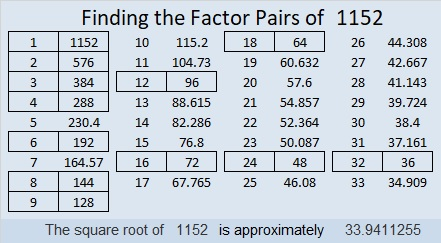The last two digits of 1152 are 52, a number divisible by 4, so 1152 can be evenly divided by 4.
1 + 1 + 5 + 2 = 9, so 1152 is divisible by 9.

It is so easy to tell if a number can be evenly divided by 4 or 9 AND it is so easy to divide by 4 or by 9. When I make a factor cake, I like to see if the current layer of the cake is divisible by 4 or by 9 before I check to see if it is divisible by a prime number.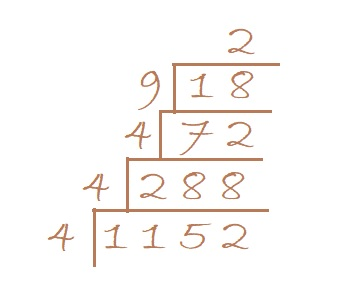From that cake, I can quickly tell that 1152 = 2⁷ × 3² by simply counting by 2’s to find the powers of 2 and 3. All the numbers being the same color doesn’t even slow me down.

I can also easily find the √1152 by taking the square root of everything on the outside of the cake:
√1152  = √(4·4) · (√4)(√9)(√2) = (4·2·3)√2 = 24√2

Since MOST square roots that can be simplified are divisible by 4, or by 9, or by both, this is a good strategy to find their square roots.

1152 is the sum of the fourteen prime numbers from 53 to 109,
and it is the sum of the twelve prime numbers from 71 to 127.

34² – 2² = 1152 so we are only 2² = 4 numbers away from the next perfect square.

1152 looks interesting when it is written in these bases:
It’s 800 in BASE 12 because 8(12²) = 1152,
242 in BASE 23 because 2(23²) + 4(23) + 2(1) = 1152
200 in BASE 24 because 2(24²) = 1152,
WW in BASE 35 (W is 32 base 10) because 32(35) + 32(1) = 32(36) = 1152, and
it’s W0 in BASE 36 because 32(36) = 1152

# 1150 Perfectly Symmetrical Factor Trees

Factor trees can look lovely if they have symmetrical branches. 1150 can make that kind of a tree:The same number can also make a more disorderly-looking tree: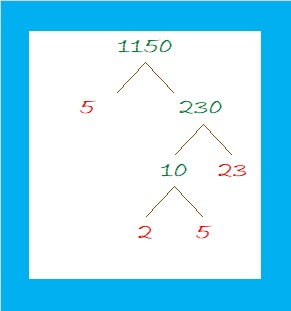All of those trees are correct factor trees. And several more can still be made for the number 1150.

What else can I tell you about that number?

• 1150 is a composite number.
• Prime factorization: 1150 = 2 × 5 × 5 × 23, which can be written 1150 = 2 × 5² × 23
• The exponents in the prime factorization are 1, 2, and 1. Adding one to each and multiplying we get (1 + 1)(2 + 1)(1 + 1) = 2 × 3 × 2 = 12. Therefore 1150 has exactly 12 factors.
• Factors of 1150: 1, 2, 5, 10, 23, 25, 46, 50, 115, 230, 575, 1150
• Factor pairs: 1150 = 1 × 1150, 2 × 575, 5 × 230, 10 × 115, 23 × 50, or 25 × 46,
• Taking the factor pair with the largest square number factor, we get √1150 = (√25)(√46) = 5√46 ≈ 33.911651150 is the hypotenuse of two Pythagorean triples:
690-920-1150 which is (3-4-5) times 230
322-1104-1150 which is (7-24-25) times 46

1150 looks interesting when it is written in a couple of different bases:
It’s 3232 in BASE 7 because 3(7³) + 2(7²) + 3(7) + 2(1) = 1150
and 6A6 in BASE 13 (A is 10 base 10) because 6(13²) + 10(13) + 6(1) = 1150

# 1144 Let’s Make a Factor Cake!

Occasionally, I’ll put a factor cake on this blog, but until today, I haven’t really shown you how to MAKE a factor cake. I made this simple gif to show you how to make a factor cake from start to finish. You can watch it over and over again if you like. Just repeatedly divide by the smallest prime number divisor.make science GIFs like this at MakeaGif

Here’s more about the number 1144:

• 1144 is a composite number.
• Prime factorization: 1144 = 2 × 2 × 2 × 11 × 13, which can be written 1144 = 2³ × 11 × 13
• The exponents in the prime factorization are 3, 1, and 1. Adding one to each and multiplying we get (3 + 1)(1 + 1)(1 + 1) = 4 × 2 × 2 = 16. Therefore 1144 has exactly 16 factors.
• Factors of 1144: 1, 2, 4, 8, 11, 13, 22, 26, 44, 52, 88, 104, 143, 286, 572, 1144
• Factor pairs: 1144 = 1 × 1144, 2 × 572, 4 × 286, 8 × 143, 11 × 104, 13 × 88, 22 × 52, or 26 × 44
• Taking the factor pair with the largest square number factor, we get √1144 = (√4)(√286) = 2√286 ≈ 33.82307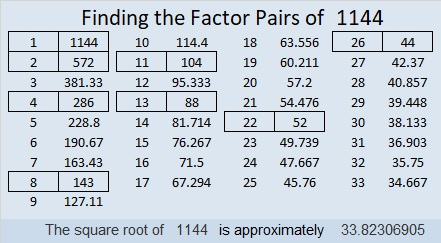1144 is obviously divisible by 11, but it isn’t so obvious that 1144 is the sum of the twenty-two prime numbers from 11 to 101.

1144 is the hypotenuse of a Pythagorean triple:
440-1056-1144 which is (5-12-13) times 88

1144 is palindrome 3223 in BASE 7 because 3(7³) + 2(7²) + 2(7) +3(1) = 1144

# 1134 Some Likely Factor Trees

I could easily make nine factor trees for 1134 using different factor pairs of 1134. However, most people aren’t likely to begin a factor tree by first dividing 1134 by 14, 18, 21, or 27. Most people will want to start with a 1-digit divisor of 1134.

Most people will also use only one color of ink or pencil lead to make a factor tree.

Here are three of 1134’s factor trees that are much more likely to be made by the average person.How easy is it to find all the prime factors in those one-color trees? You will probably think it is easier for some trees than others.

I think the tree in the middle is the easiest to read. All the prime factors are in the correct order and easy to distinguish from the composite factors in the tree. Although it is similar to using the cake method, I still like the cake method better.

Here are some facts about the number 1134:

• 1134 is a composite number.
• Prime factorization: 1134 = 2 × 3 × 3 × 3 × 3 × 7, which can be written 1134 = 2 × 3⁴ × 7
• The exponents in the prime factorization are 1, 4 and 1. Adding one to each and multiplying we get (1 + 1)(4 + 1)(1 + 1) = 2 × 5 × 2 = 20. Therefore 1134 has exactly 20 factors.
• Factors of 1134: 1, 2, 3, 6, 7, 9, 14, 18, 21, 27, 42, 54, 63, 81, 126, 162, 189, 378, 567, 1134
• Factor pairs: 1134 = 1 × 1134, 2 × 567, 3 × 378, 6 × 189, 7 × 162, 9 × 126, 14 × 81, 18 × 63, 21 × 54, or 27 × 42
• Taking the factor pair with the largest square number factor, we get √1134 = (√81)(√14) = 9√14 ≈ 33.67492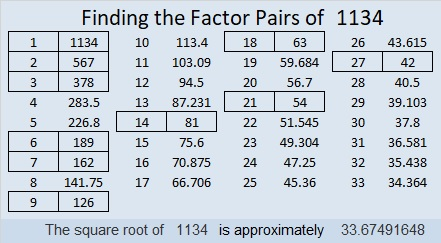1134 is the sum of four consecutive prime numbers:
277 + 281 + 283 + 293 = 1134